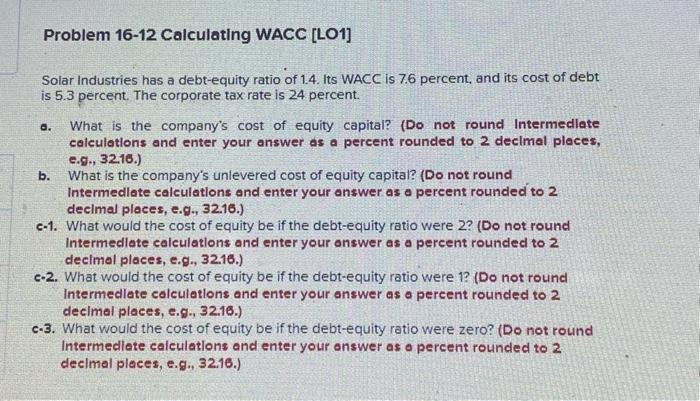Home / Expert Answers / Finance / solar-industries-has-a-debt-equity-ratio-of-1-4-its-wacc-is-7-6-percent-and-its-cost-of-debt-is-5-pa264

# (Solved): Solar Industries has a debt-equity ratio of 1.4. Its WACC is 7.6 percent, and its cost of debt is 5 ...Solar Industries has a debt-equity ratio of 1.4. Its WACC is 7.6 percent, and its cost of debt is 5.3 percent. The corporate tax rate is 24 percent. a. What is the company's cost of equity capital? (Do not round Intermedlate calculations and enter your answer as a percent rounded to 2 decimal places, e.g., 32.16.) b. What is the company's unlevered cost of equity capital? (Do not round Intermedlate calculations and enter your answer as a percent rounded to 2 decimal places, e.g., 32.16.) c-1. What would the cost of equity be if the debt-equity ratio were 2 ? (Do not round Intermedlate calculations and enter your onswer as a percent rounded to 2 decimal places, e.g., 32.16.) c-2. What would the cost of equity be if the debt-equity ratio were 1? (Do not round Intermedlate calculatlons and enter your answer as a percent rounded to 2 decimal places, e.g., 32.16.) c.3. What would the cost of equity be if the debt-equity ratio were zero? (Do not round Intermedlate calculations and enter your answer as o percent rounded to 2 declmal places, e.g., 32.16.)

We have an Answer from Expert